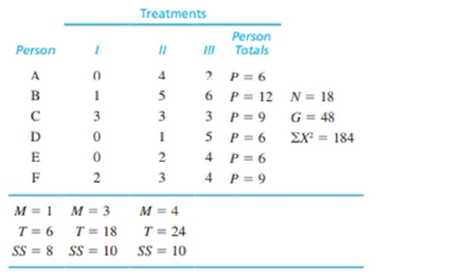Chapter 13, Problem 2REEssentials of Statistics for the B...

8th Edition
Frederick J Gravetter + 1 other
ISBN: 9781133956570

Solutions

Chapter
SectionEssentials of Statistics for the B...

8th Edition
Frederick J Gravetter + 1 other
ISBN: 9781133956570
Textbook Problem

The following data were obtained from a repeated- measures study comparing three treatment conditions.a. Do the data indicate significant differences among the three treatments? Test at the .05 level of significance.b. Calculate try to measure the size of the effect.c. Write a sentence demonstrating how the outcome of the hypothesis test and the measure of effect size would appear in a research report.

a.

To determine
Whether there are significant mean difference among the three conditions using ANOVA at α=0.05.

Explanation

Given info: The data is tabulated as:

 Treatment Person total N=18G=48∑X2=184 M=1T=6SS=8 M=3T=18SS=10 M=4T=24SS=10 p=6 p=12 p=9 p=6 p=6 p=9

Calculation:

Step 1:

Null hypothesis:

H0:μ1=μ2=μ3

Alternate hypothesis

Ha: At least one of treatment mean is different.

Step 2: Degrees of freedom and critical value: (First stage)

dftotal=N-1=17

And,

dfbetween=k1=2

So,

dfwithin=dftotaldfbetweentreatment=172=15

Now,

SStotal=X2G2N=18448218=56

Similarly,

SSwithintreatment=SSinsideeachtreatment=8+10+10=28

So,

SSbetween=5628=28

At second stage compute SSerror,SSbetweensubject:

b.

To determine

To find: The value of η2 for the given information.

c.

To determine

To write: The results in form of a report.

Still sussing out bartleby?

Check out a sample textbook solution.

See a sample solution

The Solution to Your Study Problems

Bartleby provides explanations to thousands of textbook problems written by our experts, many with advanced degrees!

Get Started

In Exercises 7-12, refer to the following figure. 9. Which points have negative y-coordinates?

Applied Calculus for the Managerial, Life, and Social Sciences: A Brief Approach

How much work is done when a hoist lifts a 200-kg rock to a height of 3 m?

Single Variable Calculus: Early Transcendentals, Volume I

In Exercises 110, evaluate the expression. 15!

Finite Mathematics for the Managerial, Life, and Social Sciences

The area of the region bounded by , , and r = sec θ is:

Study Guide for Stewart's Single Variable Calculus: Early Transcendentals, 8th